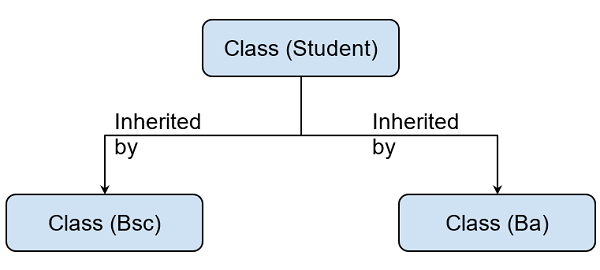# Example of Hierarchical Inheritance in Python (2)

Here, we are going to learn about the hierarchical inheritance in Python with an example.
Submitted by Shivang Yadav, on February 19, 2021

Program Statement:

We will create a class named student which is inherited by two classes Bsc and Ba. Then we have used the get method to get input of information from the user. And then print the details using the print method.Classes and members:

• Class - student
• Method - getStudentInfo() : Gets input from user for variables of the class.
• Method - putStudent() : prints student information.
• Variable - rollNo : stores student's roll number.
• Variable - name : stores student's name.
• Class - Bsc
• Method - getBsc() : Gets input from user for variables of the class.
• Method - putBsc() : prints Bsc information and calls putStudent.
• Variable - p : stores student's physics marks.
• Variable - c : stores student's chemistry marks.
• Variable - m : stores student's maths marks.
• Class Ba
• Method - getBa() : Gets input from user for variables of the class.
• Method - putBa() : prints Ba information and calls putStudent.
• Variable - h : stores student's history marks.
• Variable - g : stores student's geography marks.
• Variable - e : stores student's economics marks.

Python program to illustrate Hierarchical Inheritance

```class Student:
def getStudentInfo(self):
self.__rollno=input("Roll Number: ")
self.__name=input("Name: ")

def PutStudent(self):
print("Roll Number : ", self.__rollno,"Name : ", self.__name)

class Bsc(Student):
def GetBsc(self):
self.getStudentInfo()
self.__p=int(input("Physics Marks: "))
self.__c = int(input("Chemistry Marks: "))
self.__m = int(input("Maths Marks: "))

def PutBsc(self):
self.PutStudent()
print("Marks is Subjects ", self.__p,self.__c,self.__m)

class Ba(Student):
def GetBa(self):
self.getStudentInfo()
self.__h = int(input("History Marks: "))
self.__g = int(input("Geography Marks: "))
self.__e = int(input("Economic Marks: "))

def PutBa(self):
self.PutStudent()
print("Marks is Subjects ", self.__h,self.__g,self.__e)

print("Enter Bsc Student's details")
student1=Bsc()
student1.GetBsc()
student1.PutBsc()

print("Enter Ba Student's details")
student2=Ba()
student2.GetBa()
student2.PutBa()
```

Output:

```Enter Bsc Student's details
Roll Number: 004
Name: john
Physics Marks: 78
Chemistry Marks: 67
Maths Marks: 89
Roll Number :  004 Name :  john
Marks is Subjects  78 67 89
Enter Ba Student's details
Roll Number: jane      0021
Name: jane
History Marks: 43
Geography Marks: 76
Economic Marks: 98
Roll Number :  0021 Name :  jane
Marks is Subjects  43 76 98
```

Preparation

What's New

Top Interview Coding Problems/Challenges!